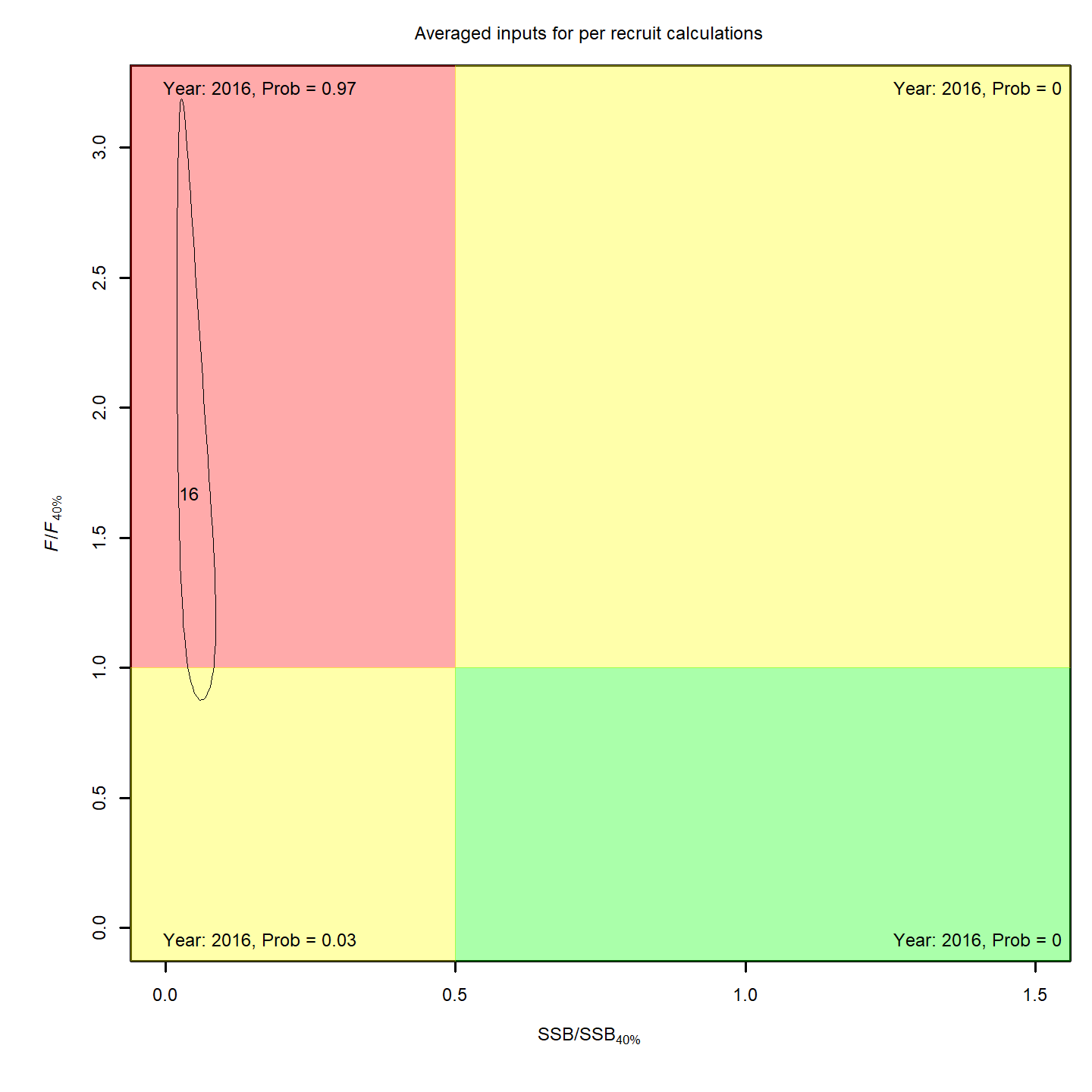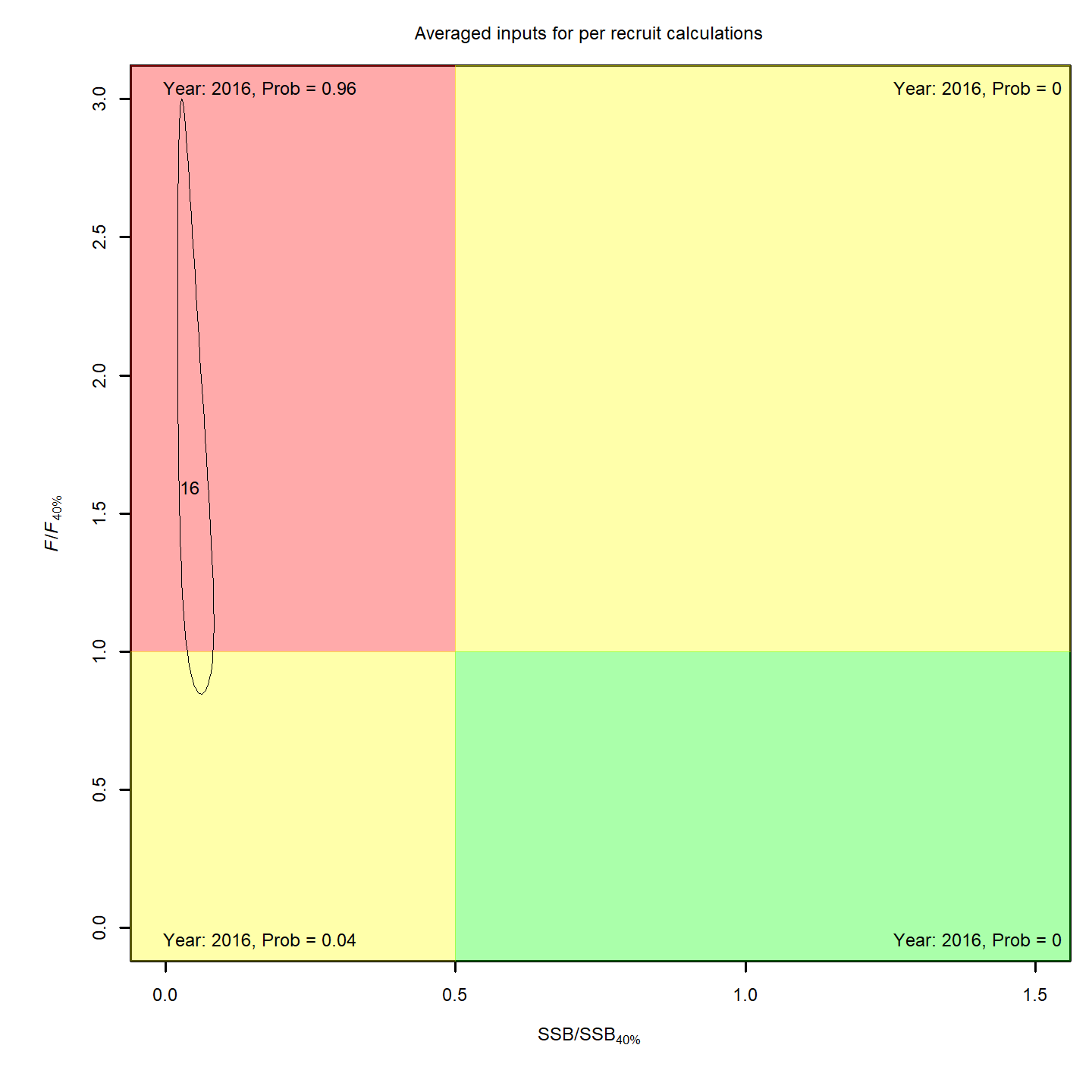In this vignette we walk through an example using the wham (WHAM = Woods Hole Assessment Model) package to run a state-space age-structured stock assessment model. WHAM is a generalization of code written for Miller et al. (2016) and Xu et al. (2018), and in this example we apply WHAM to the same stock, Southern New England / Mid-Atlantic Yellowtail Flounder.

This is the 6th WHAM example, which blends aspects from Ex 1, Ex 2, and Ex 5. We assume you already have wham installed. If not, see the Introduction. The simpler 1st example is available as a R script and vignette.

As in example 1:

• Stock: Southern New England-Mid Atlantic (SNEMA) yellowtail flounder
• Data: 1973-2011, 1 fishery and 2 indices
• Age compositions: logistic normal, without pooling zero observations (input$data$age_comp_model_fleets = 7 and input$data$age_comp_model_indices = 7)
• Selectivity: age-specific

As in example 2:

• Beverton-Holt recruitment (recruit_model = 3)
• Environmental covariate (ecov) modeled as an AR1 process (ecov$process_model = 'ar1') • Compare with and w/o ecov having a “limiting” (carrying capacity) effect on recruitment (ecov$how = 2)

As in example 5:

• Environmental covariate: Gulf Stream Index (GSI)
• 2D AR1 deviations by age and year (random effects)

Example 6 highlights WHAM’s options for treating the yearly transitions in numbers-at-age (i.e. survival):

1. Deterministic (as in statistical catch-at-age models, recruitment in each year estimated as independent fixed effect parameters)
2. Recruitment deviations (from Bev-Holt expectation) are random effects
• independent
• AR1 deviations by year (autocorrelated)
3. “Full state-space model” (survival of all ages are random effects)
• independent
• AR1 deviations by age
• AR1 deviations by year
• 2D AR1 deviations by age and year

Open R and load the wham package:

# devtools::install_github("timjmiller/wham", dependencies=TRUE)
library(tidyverse)
library(wham)

For a clean, runnable .R script, look at ex6_NAA.R in the example_scripts folder of the wham package install:

wham.dir <- find.package("wham")
file.path(wham.dir, "example_scripts")

You can run this entire example script with:

write.dir <- "choose/where/to/save/output" # otherwise will be saved in working directory
source(file.path(wham.dir, "example_scripts", "ex6_NAA.R"))

Let’s create a directory for this analysis:

# choose a location to save output, otherwise will be saved in working directory
write.dir <- "choose/where/to/save/output"
dir.create(write.dir)
setwd(write.dir)

We need the same ASAP data file as in example 1, and the environmental covariate (Gulf Stream Index, GSI). Let’s copy ex1_SNEMAYT.dat and GSI.csv to our analysis directory:

wham.dir <- find.package("wham")
file.copy(from=file.path(wham.dir,"extdata","ex1_SNEMAYT.dat"), to=write.dir, overwrite=FALSE)
file.copy(from=file.path(wham.dir,"extdata","GSI.csv"), to=write.dir, overwrite=FALSE)

Confirm you are in the correct directory and it has the required data files:

list.files()

Read the ASAP3 .dat file into R and convert to input list for wham:

asap3 <- read_asap3_dat("ex1_SNEMAYT.dat")

Load the environmental covariate (Gulf Stream Index, GSI) data into R:

env.dat <- read.csv("GSI.csv", header=T)
#>   year        GSI
#> 1 1954  0.8876748
#> 2 1955  0.3024170
#> 3 1956 -1.2004947
#> 4 1957 -0.2408031
#> 5 1958 -0.7806940
#> 6 1959 -1.3218938

As in example 5, the GSI data file does not have a standard error estimate, either for each yearly observation or one overall value. In such a case, WHAM can estimate the observation error for the environmental covariate, either as one overall value, $$\sigma_{GSI}$$, or yearly values as random effects, $$\mathrm{log}\sigma_{{GSI}_y} \sim \mathcal{N}(\mathrm{log}\sigma_{GSI}, \sigma^2_{\sigma_{GSI}})$$. In this example we choose the simpler option and estimate one observation error parameter, shared across years.

## 2. Specify models

Now we specify several models with different options for the numbers-at-age (NAA) transitions, i.e. survival:

df.mods <- data.frame(NAA_cor = c('---','iid','ar1_y','iid','ar1_a','ar1_y','2dar1','iid','ar1_y','iid','ar1_a','ar1_y','2dar1'),
NAA_sigma = c('---',rep("rec",2),rep("rec+1",4),rep("rec",2),rep("rec+1",4)),
GSI_how = c(rep(0,7),rep(2,6)), stringsAsFactors=FALSE)
n.mods <- dim(df.mods)
df.mods$Model <- paste0("m",1:n.mods) df.mods <- df.mods %>% select(Model, everything()) # moves Model to first col Look at the model table: df.mods #> Model NAA_cor NAA_sigma GSI_how #> 1 m1 --- --- 0 #> 2 m2 iid rec 0 #> 3 m3 ar1_y rec 0 #> 4 m4 iid rec+1 0 #> 5 m5 ar1_a rec+1 0 #> 6 m6 ar1_y rec+1 0 #> 7 m7 2dar1 rec+1 0 #> 8 m8 iid rec 2 #> 9 m9 ar1_y rec 2 #> 10 m10 iid rec+1 2 #> 11 m11 ar1_a rec+1 2 #> 12 m12 ar1_y rec+1 2 #> 13 m13 2dar1 rec+1 2 ## 3. Numbers-at-age options To specify the options for modeling NAA transitions, include an optional list argument, NAA_re, to the prepare_wham_input function (see the prepare_wham_input help page). ASAP3 does not estimate random effects, and therefore these options are not specified in the ASAP data file. By default (NAA_re is NULL or not included), WHAM fits a traditional statistical catch-at-age model (NAA = predicted NAA for all ages, i.e. survival is deterministic). To fit a state-space model, we must specify NAA_re. NAA_re is a list with the following entries: • $sigma: Which ages allow deviations from pred_NAA? Common options are specified with strings.
• "rec": Recruitment deviations are random effects, survival of all other ages is deterministic
• "rec+1": Survival of all ages is stochastic (“full state space model”), with 2 estimated $$\sigma_a$$, one for recruitment and one shared among other ages
• $cor: Correlation structure for the NAA deviations. Options are: • "iid": NAA deviations vary by year and age, but uncorrelated. • "ar1_a": NAA deviations correlated by age (AR1). • "ar1_y": NAA deviations correlated by year (AR1). • "2dar1": NAA deviations correlated by year and age (2D AR1, as for $$M$$ in example 5). Alternatively, you can specify a more complex NAA_re$sigma by entering a vector with length equal to the number of ages, where each entry points to the $$\sigma_a$$ to use for that age. For example, NAA_re$sigma = c(1,2,2,3,3,3) will estimate three $$\sigma_a$$, with recruitment (age-1) deviations having their own $$\sigma_R$$, ages 2-3 sharing $$\sigma_2$$, and ages 4-6 sharing $$\sigma_3$$. For example, to fit model m1 (SCAA): NAA_re <- NULL # or simply leave out of call to prepare_wham_input To fit model m3, recruitment deviations are correlated random effects: NAA_re <- list(sigma="rec", cor="ar1_y") And to fit model m7, numbers at all ages are random effects correlated by year AND age: NAA_re <- list(sigma="rec+1", cor="2dar1") ## 4. Linking recruitment to an environmental covariate (GSI) As described in example 2, the environmental covariate options are fed to prepare_wham_input as a list, ecov. This example differs from example 2 in that: • ecov$logsigma = "est_1" estimates the GSI observation error ($$\sigma_{GSI}$$, one overall value for all years). The other option is "est_re" to allow the GSI observation error to have yearly fluctuations (random effects). The Cold Pool Index in example 2 had yearly observation errors given, so this was not necessary.
• ecov$how = 0 estimates the GSI time-series model (AR1) for models without a GSI-Recruitment effect, in order to compare AIC with models that do include the effect. Setting ecov$how = 2 specifies that the GSI affects the Beverton-Holt $$b$$ parameter (“limiting” / carrying capacity effect).
• ecov$link_model = "linear" specifies a linear model linking GSI to $$b$$. For example, the ecov list for models m8-m13 with the linear GSI-$$b$$ effect:  # example for model m3 ecov <- list( label = "GSI", mean = as.matrix(env.dat$GSI),
logsigma = 'est_1', # estimate obs sigma, 1 value shared across years
year = env.dat$year, use_obs = matrix(1, ncol=1, nrow=dim(env.dat)), # use all obs (=1) lag = 1, # GSI in year t affects recruitment in year t+1 process_model = "ar1", # GSI modeled as AR1 (random walk would be "rw") where = "recruit", # GSI affects recruitment how = 2, # limiting link_model = "linear") # linear GSI-Recruitment effect Note that you can set ecov = NULL to fit the model without environmental covariate data, but here we fit the ecov data even for models without the GSI effect on recruitment (m1-m7) so that we can compare them via AIC (need to have the same data in the likelihood). We accomplish this by setting ecov$how = 0 and ecov$process_model = "ar1". ## 5. Run all models All models use the same options for expected recruitment (Beverton-Holt stock-recruit function) and selectivity (age-specific, with one or two ages fixed at 1). for(m in 1:n.mods){ NAA_list <- list(cor=df.mods[m,"NAA_cor"], sigma=df.mods[m,"NAA_sigma"]) if(NAA_list$sigma == '---') NAA_list = NULL

ecov <- list(
label = "GSI",
mean = as.matrix(env.dat$GSI), logsigma = 'est_1', # estimate obs sigma, 1 value shared across years year = env.dat$year,
use_obs = matrix(1, ncol=1, nrow=dim(env.dat)), # use all obs (=1)
lag = 1, # GSI in year t affects Rec in year t + 1
process_model = 'ar1', # "rw" or "ar1"
where = "recruit", # GSI affects recruitment
how = df.mods$GSI_how[m], # 0 = no effect (but still fit Ecov to compare AIC), 2 = limiting link_model = "linear") input <- prepare_wham_input(asap3, recruit_model = 3, # Bev Holt recruitment model_name = "Ex 6: Numbers-at-age", selectivity=list(model=rep("age-specific",3), re=rep("none",3), initial_pars=list(c(0.5,0.5,0.5,1,1,0.5),c(0.5,0.5,0.5,1,0.5,0.5),c(0.5,1,0.5,0.5,0.5,0.5)), fix_pars=list(c(4,5),4,2)), NAA_re = NAA_list, ecov=ecov) # overwrite age comp model (all models use logistic normal) input$data$age_comp_model_indices = rep(7, input$data$n_indices) input$data$age_comp_model_fleets = rep(7, input$data$n_fleets) input$data$n_age_comp_pars_indices = rep(1, input$data$n_indices) input$data$n_age_comp_pars_fleets = rep(1, input$data$n_fleets) input$par$index_paa_pars = rep(0, input$data$n_indices) input$par$catch_paa_pars = rep(0, input$data$n_fleets) input$map = input$map[!(names(input$map) %in% c("index_paa_pars", "catch_paa_pars"))]

# Fit model
mod <- fit_wham(input, do.retro=T, do.osa=F)

# Save model
saveRDS(mod, file=paste0(df.mods$Model[m],".rds")) # If desired, plot output in new subfolder # plot_wham_output(mod=mod, dir.main=file.path(getwd(),df.mods$Model[m]), out.type='html')

# If desired, do projections
# mod_proj <- project_wham(mod)
# saveRDS(mod_proj, file=paste0(df.mods$Model[m],"_proj.rds")) } ## 6. Compare models Collect all models into a list. mod.list <- paste0(df.mods$Model,".rds")
mods <- lapply(mod.list, readRDS)

Get model convergence and stats.

opt_conv = 1-sapply(mods, function(x) x$opt$convergence)
ok_sdrep = sapply(mods, function(x) if(x$na_sdrep==FALSE & !is.na(x$na_sdrep)) 1 else 0)
df.mods$conv <- as.logical(opt_conv) df.mods$pdHess <- as.logical(ok_sdrep)
df.mods$runtime <- sapply(mods, function(x) x$runtime)
df.mods$NLL <- sapply(mods, function(x) round(x$opt$objective,3)) df.aic <- as.data.frame(compare_wham_models(mods, sort=FALSE, calc.rho=T)$tab)
df.aic$AIC[df.mods$pdHess==FALSE] <- NA
minAIC <- min(df.aic$AIC, na.rm=T) df.aic$dAIC <- round(df.aic$AIC - minAIC,1) df.mods <- cbind(df.mods, df.aic) rownames(df.mods) <- NULL Look at results table. df.mods ## 7. Results Two models had very similar AIC and were overwhelmingly supported relative to the other models (bold in table below): m11 (all NAA are random effects with correlation by age, GSI-Recruitment effect) and m13 (all NAA are random effects with correlation by age and year, GSI-Recruitment effect). The SCAA and state-space models with independent NAA deviations had the lowest runtime. Estimating NAA deviations only for age-1, i.e. recruitment as random effects, broke the Hessian sparseness, making models m2, m3, m8, and m9 the slowest. Adding correlation structure to the NAA deviations increased runtime by a factor of 2-3 (comparing models m4-m7 and m10-m13). In the table, I have highlighted models which converged and successfully inverted the Hessian to produce SE estimates for all (fixed effect) parameters. WHAM stores this information in mod$na_sdrep (should be FALSE), mod$sdrep$pdHess (should be TRUE), and mod$opt$convergence (should be 0). See stats::nlminb() and TMB::sdreport() for details.

We leave the AIC for model m1 blank because comparing models in which parameters (here, recruitment deviations) are estimated as fixed effects versus random effects is messy and marginal AIC is not appropriate. Still, we include m1 to show 1) that WHAM can fit this NAA option, and 2) the poor retrospective pattern in the status quo assessment.

Model NAA_cor NAA_sigma GSI_how Converged Pos def Hessian Runtime (min) NLL $$\Delta AIC$$ AIC $$\rho_{R}$$ $$\rho_{SSB}$$ $$\rho_{\overline{F}}$$
m1 TRUE TRUE 0.36 -721.490 1.941 -0.052 -0.052
m2 iid rec TRUE TRUE 3.71 -608.670 332 -1065.3 3.290 -0.068 -0.024
m3 ar1_y rec TRUE TRUE 3.54 -622.626 306 -1091.3 2.565 -0.067 -0.021
m4 iid rec+1 TRUE TRUE 0.74 -757.815 35.7 -1361.6 0.373 0.062 -0.048
m5 ar1_a rec+1 TRUE FALSE 1.77 -768.243 NA NA 0.219 0.060 -0.054
m6 ar1_y rec+1 TRUE TRUE 2.19 -763.434 26.4 -1370.9 0.380 0.031 -0.012
m7 2dar1 rec+1 TRUE TRUE 2.39 -771.570 12.2 -1385.1 0.218 0.034 -0.029
m8 iid rec Limiting TRUE TRUE 3.76 -623.722 303.9 -1093.4 1.149 -0.089 -0.001
m9 ar1_y rec Limiting TRUE TRUE 3.94 -626.705 299.9 -1097.4 1.662 -0.061 -0.024
m10 iid rec+1 Limiting TRUE TRUE 0.77 -767.957 17.4 -1379.9 0.242 0.007 -0.010
m11 ar1_a rec+1 Limiting TRUE TRUE 1.73 -777.633 0 -1397.3 0.147 0.041 -0.038
m12 ar1_y rec+1 Limiting TRUE TRUE 2.31 -769.801 15.7 -1381.6 0.228 0.009 0.003
m13 2dar1 rec+1 Limiting TRUE TRUE 2.49 -778.482 0.3 -1397 0.116 0.023 -0.019

#### Estimated NAA deviations

• All models estimated positive recruitment (age-1) deviations in the 1970s and 80s (red), and primarily negative deviations since 1990 (blue).
• Models with all NAA as random effects estimated less extreme recruitment deviations (lighter red and blue for age 1).
• Models with a GSI-Recruitment link had less trend in recruitment deviations, because the GSI effect on expected recruitment accounted for this trend.

Estimated survival deviations by age (y-axis) and year (x-axis) for all models: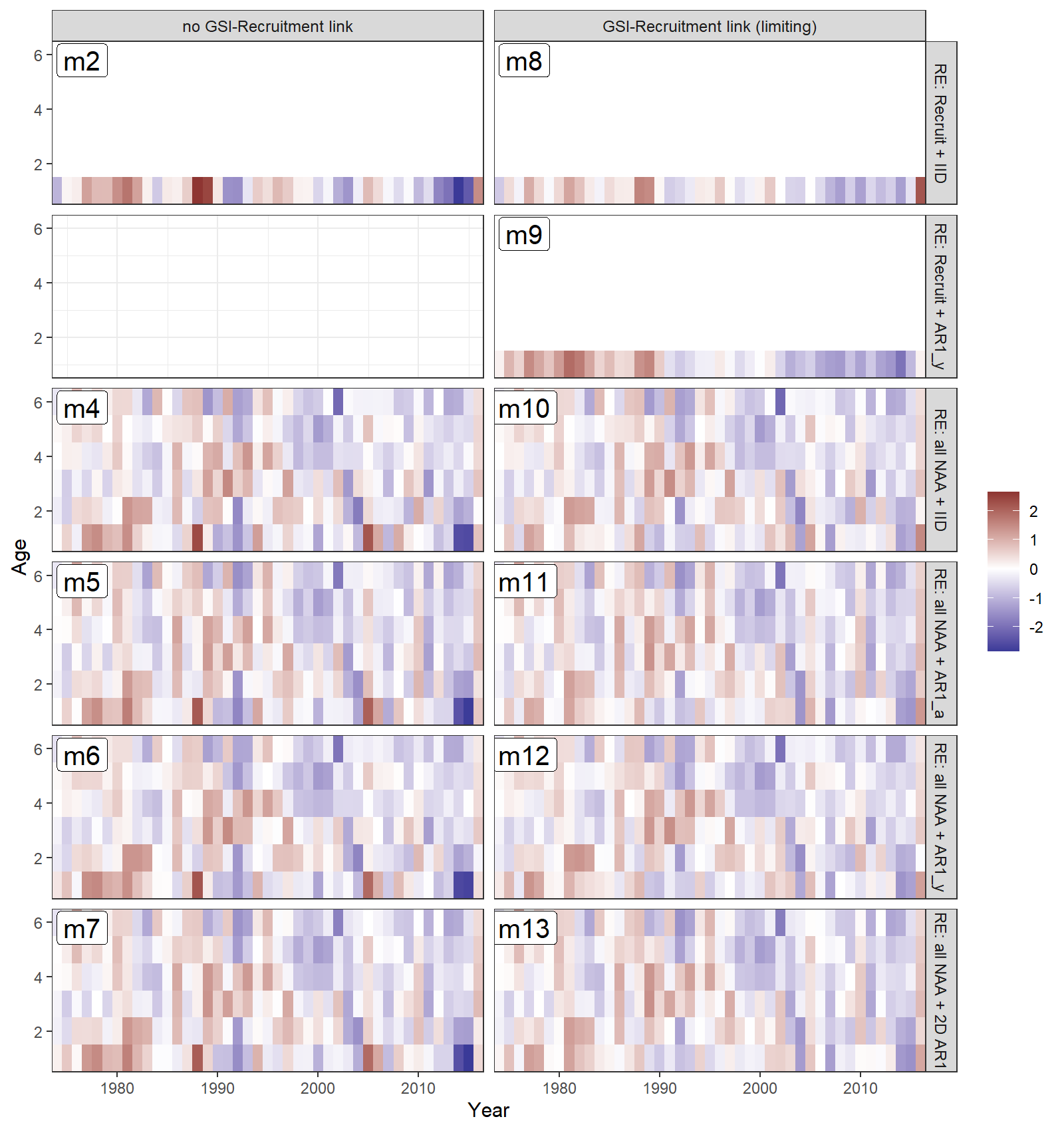#### Retrospective patterns

The base model, m1, had a severe retrospective pattern for recruitment ($$\rho_R$$ = 1.94). The full state-space model effectively alleviated this, whether or not a GSI-Recruitment effect was included ($$\rho_R$$ for m4-7 and m10-13 all below 0.38). Adding a GSI-Recruitment link to the state-space models further reduced $$\rho_R$$, and also reduced $$\rho_{SSB}$$ and $$\rho_{\overline{F}}$$.

Comparing m11 and m13, the two best models with similar AIC, m13 had lower retrospective patterns ($$\rho_R$$, $$\rho_{SSB}$$, and $$\rho_{\overline{F}}$$).

The plots below compare retrospective patterns from the base model (m1, left) to those from the full model (m13, right).

###### Numbers-at-age, NAA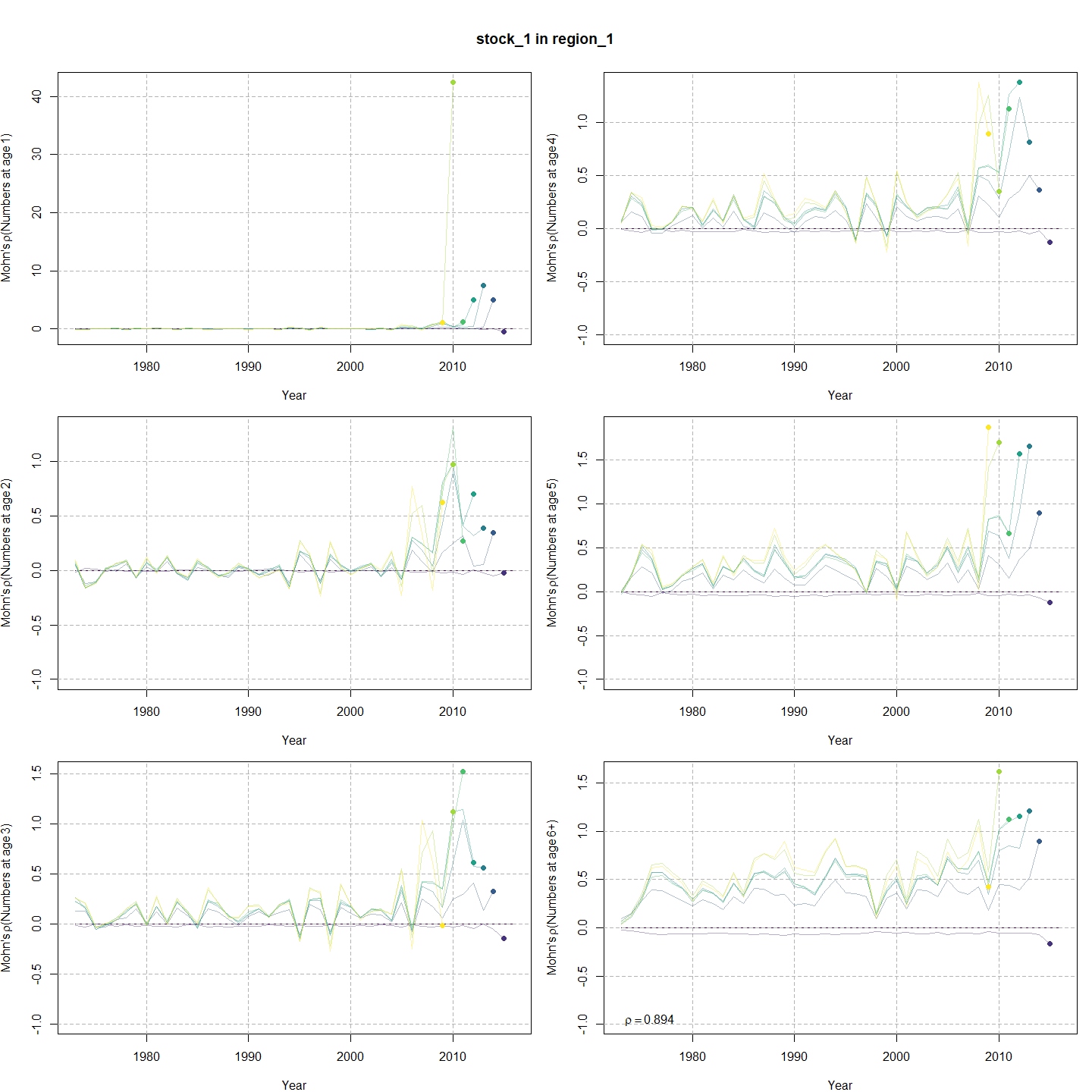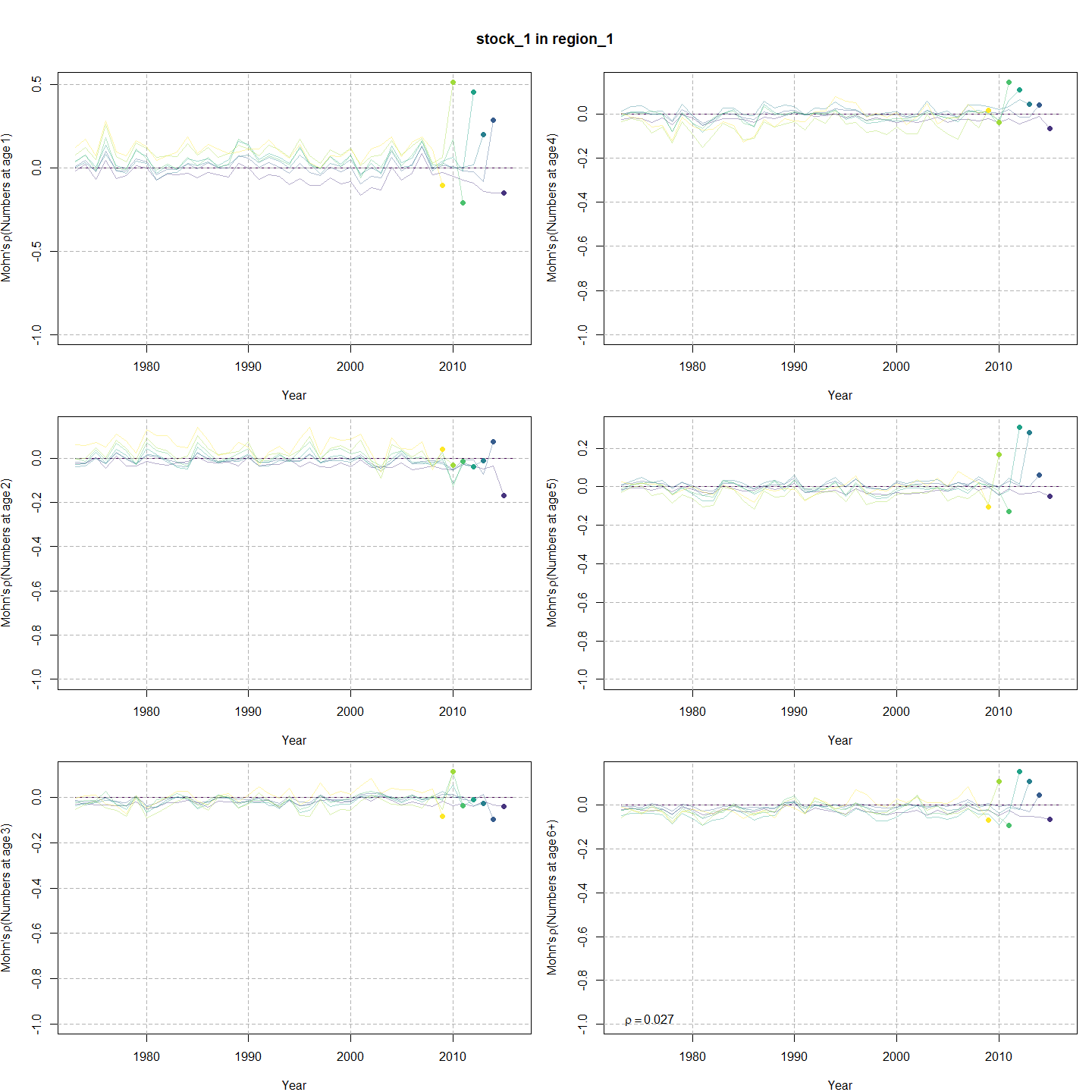###### Spawning stock biomass, SSB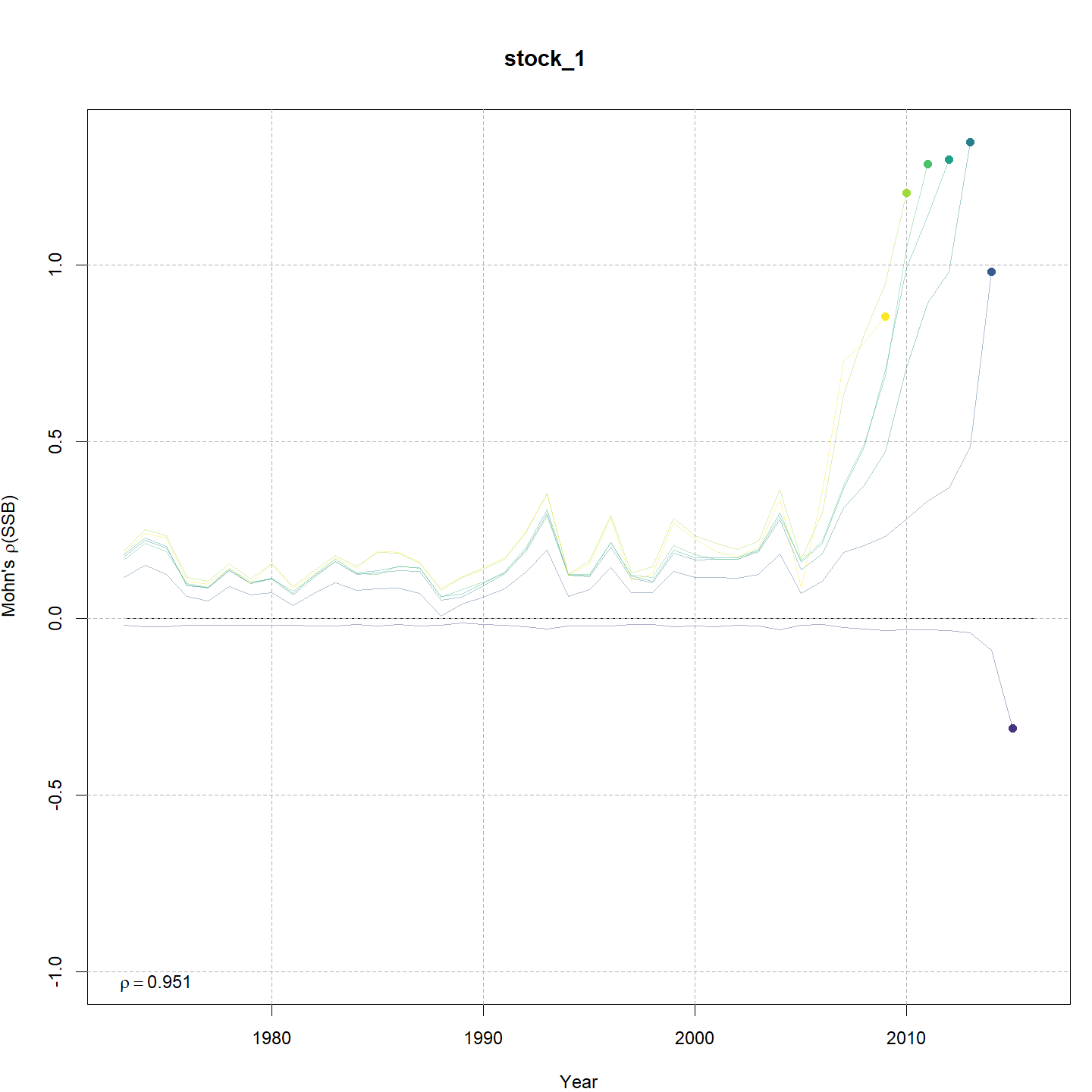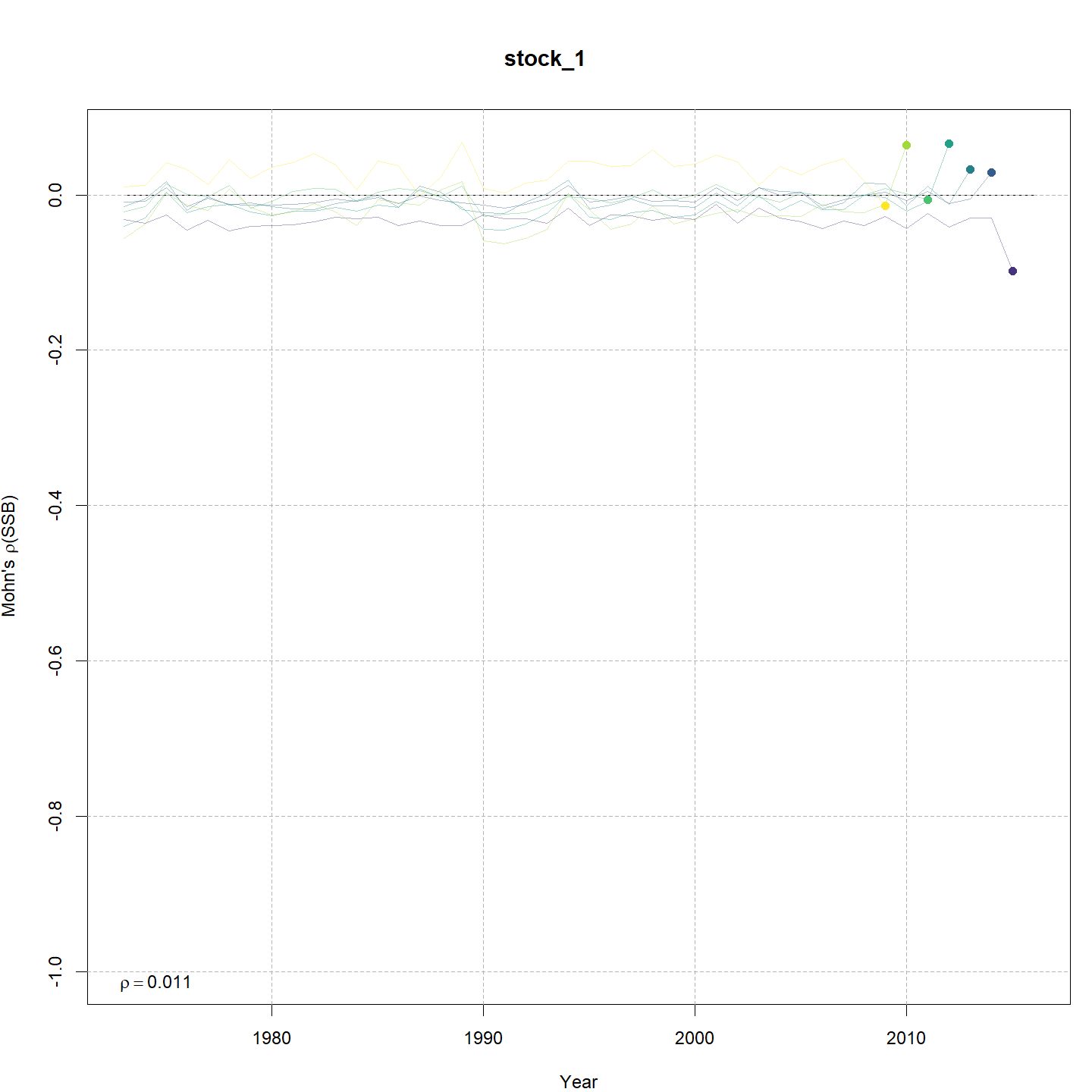###### Fishing mortality, F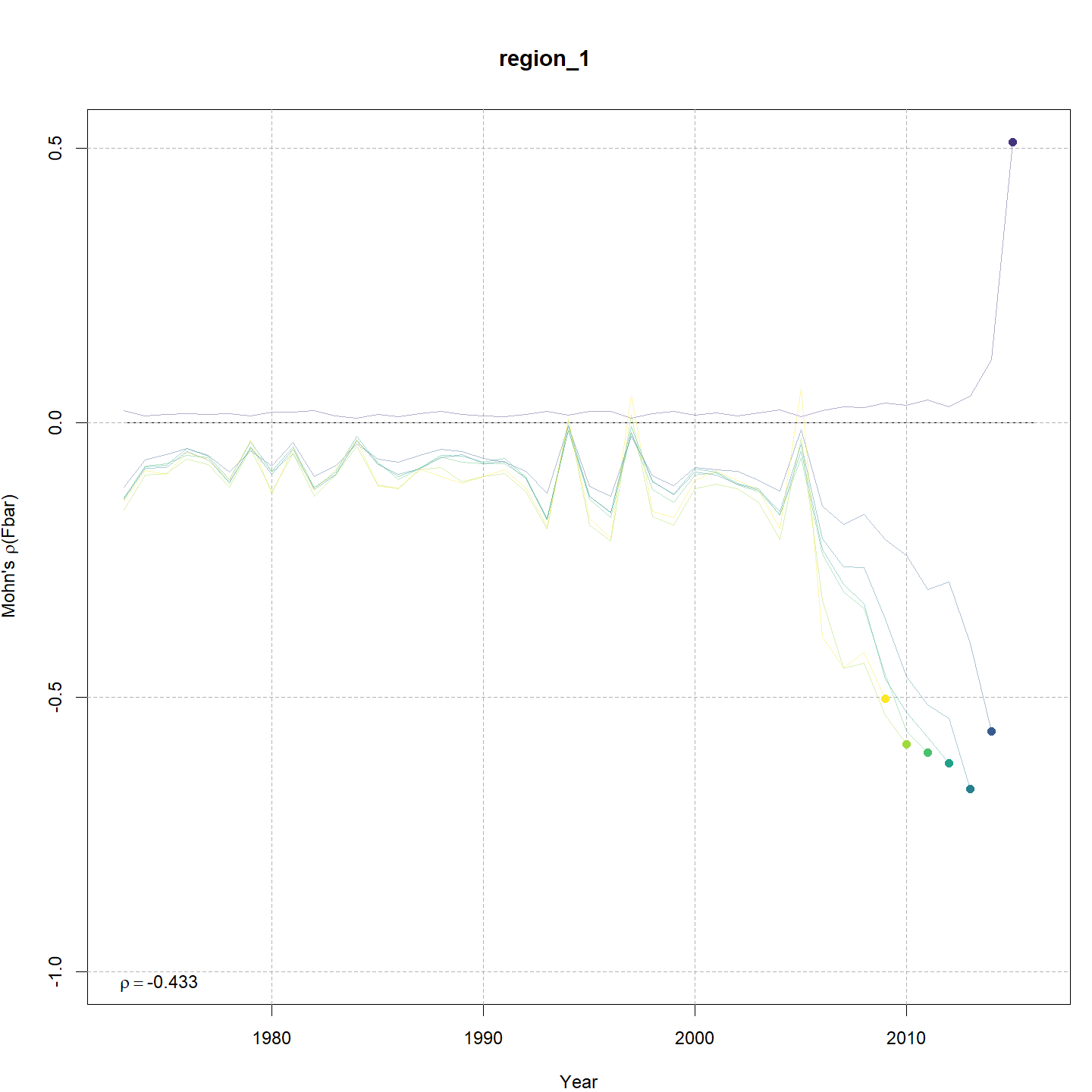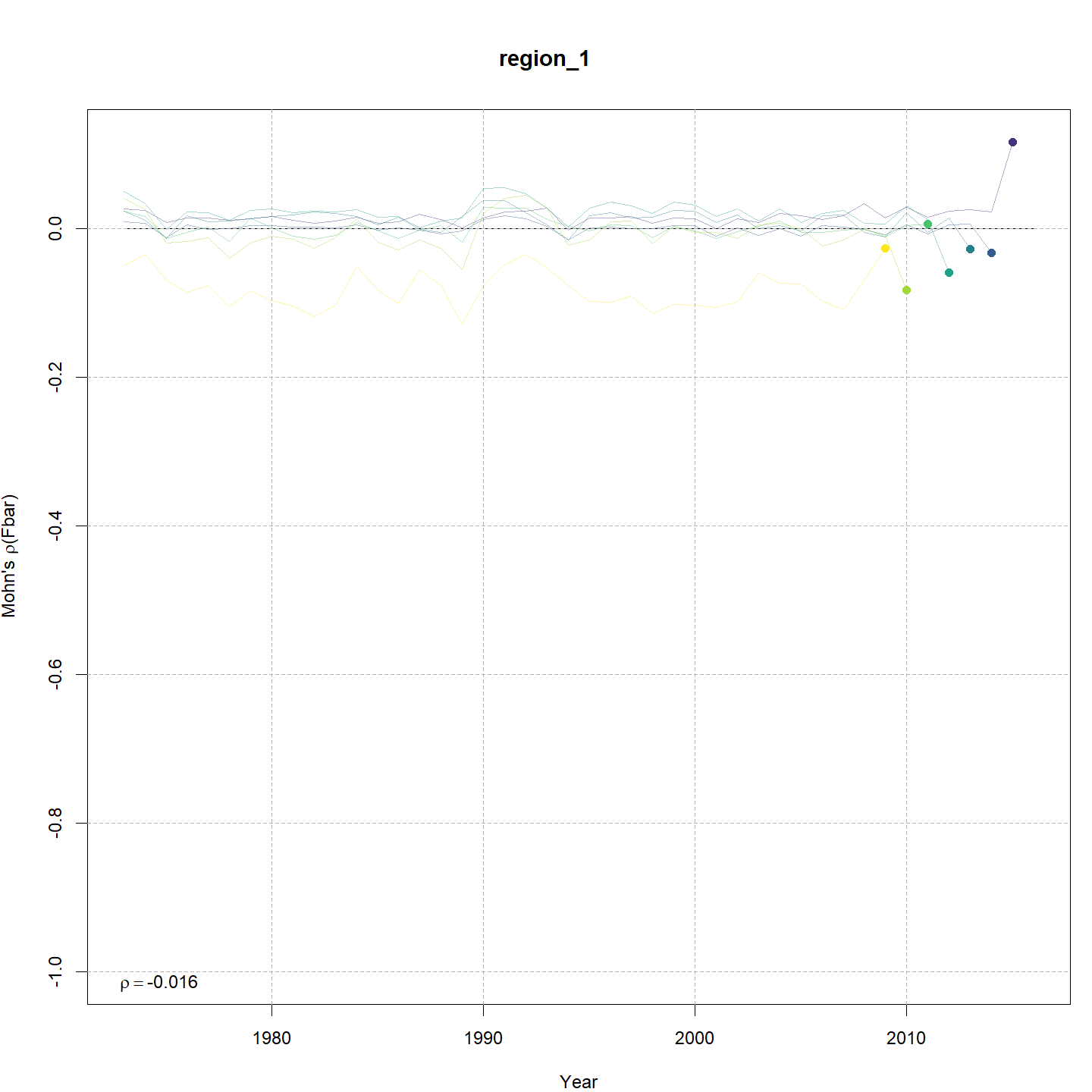#### Estimated GSI

Models with a GSI-Recruitment link estimated higher observation error and more smoothing of the GSI than those without. Compare the confidence intervals for m7 (left, no GSI-Recruitment link), to those for m13 (right, with GSI-Recruitment link).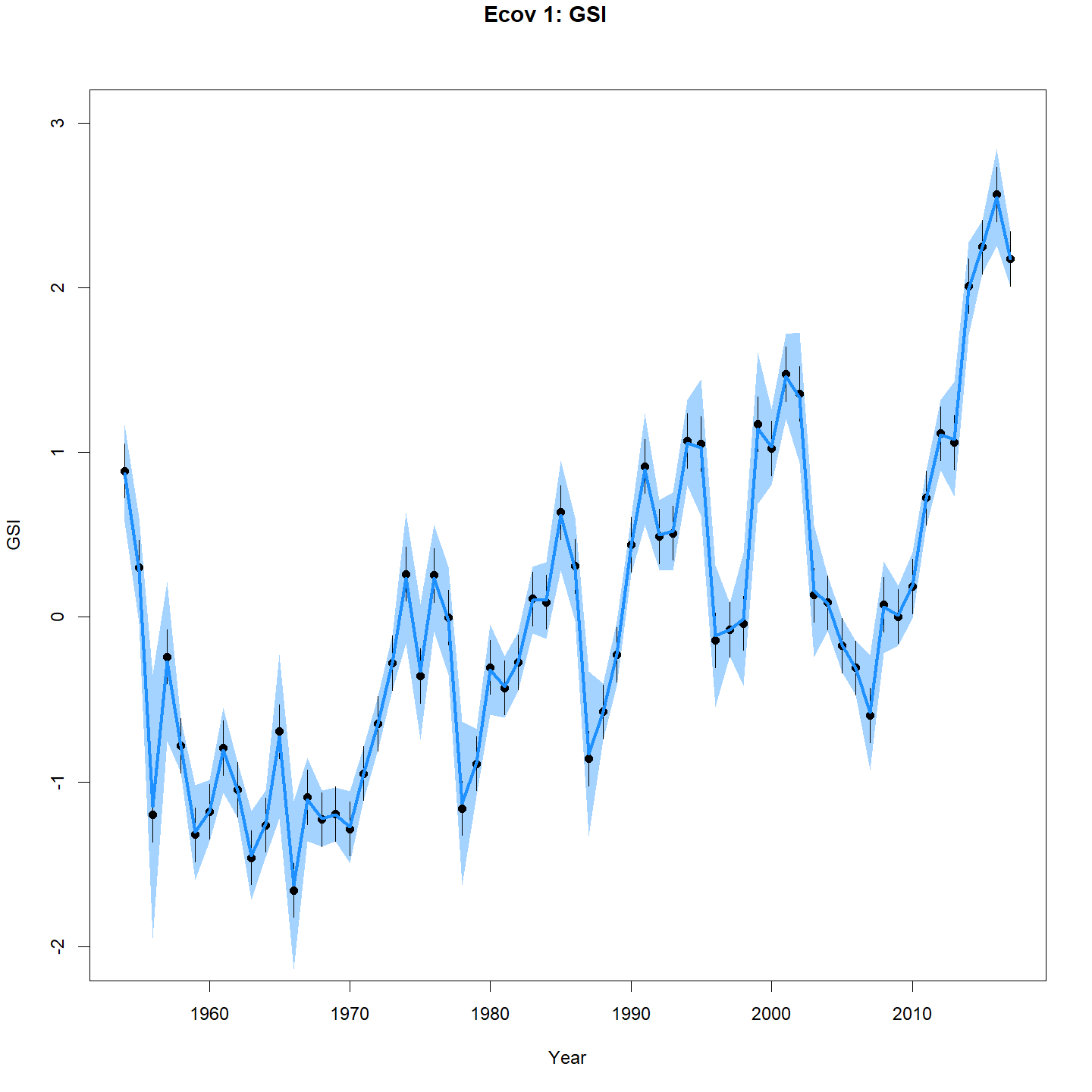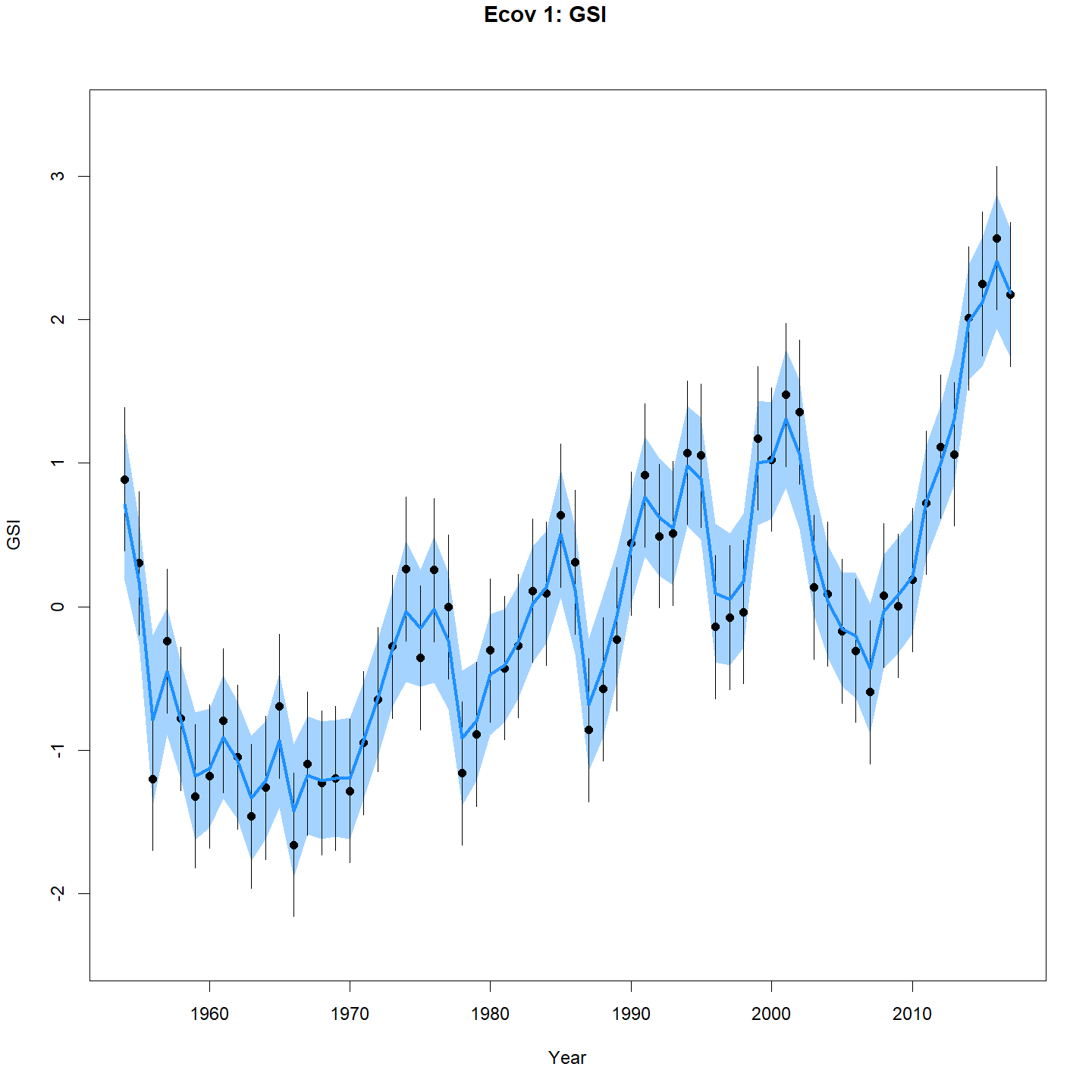#### Stock status

The state-space models, with or without the GSI-Recruitment link, estimated substantially different trends in $$SSB$$ and $$F$$ compared to the base model. Left: $$SSB$$ and $$F$$ trends from the base model, m1. Right: $$SSB$$ and $$F$$ trends from the state-space model without GSI effect, m7.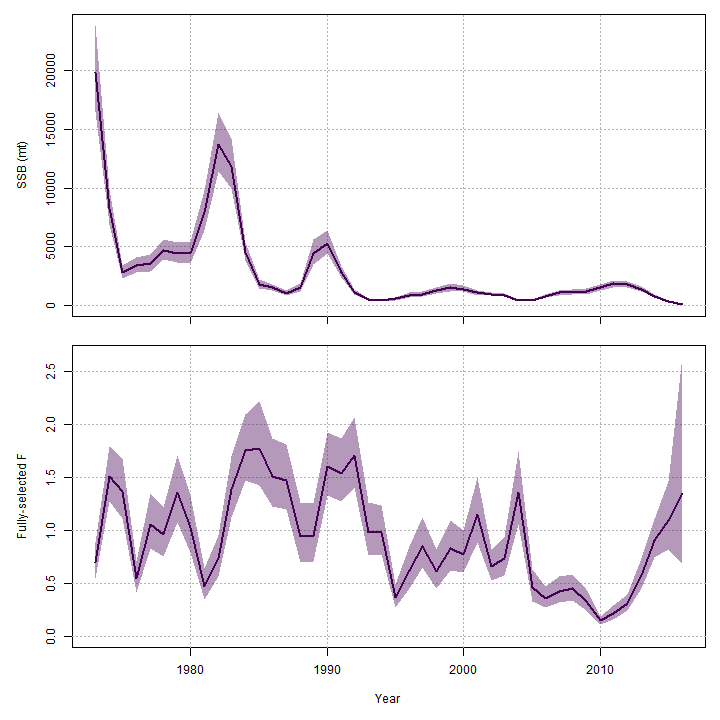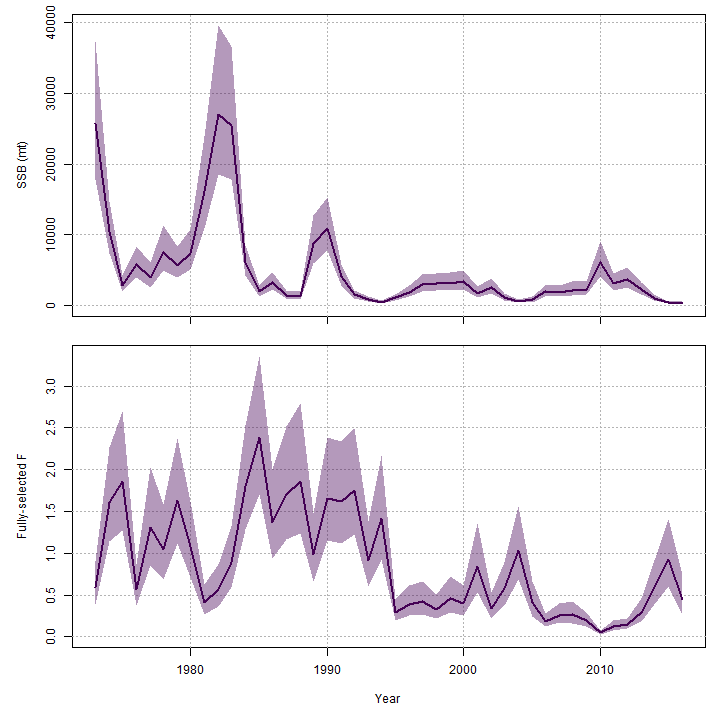Adding the GSI-Recruitment link to the state-space model estimated a lower probability of the stock being overfished in the final year, 2016 (m7 w/o GSI, 66%, left; m13 w/ GSI, 10%, right).